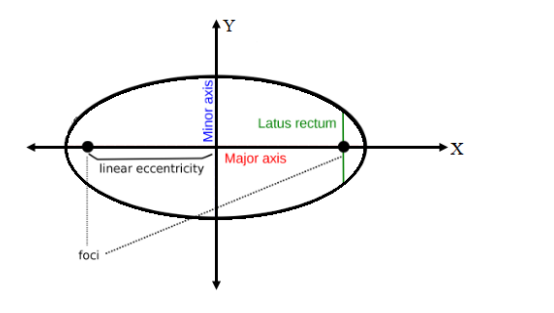# Find the eccentricity of an ellipse whose latus rectum is one half of its

Question:

Find the eccentricity of an ellipse whose latus rectum is one half of its minor axis.

Solution:Let the equation of the required ellipse is

$\frac{x^{2}}{a^{2}}+\frac{y^{2}}{b^{2}}=1$  ............(i)

It is given that

Length of Latus Rectum $=\frac{1}{2}$ minor Axis

We know that,

Length of Latus Rectum $=\frac{2 b^{2}}{a}$

and Length of Minor Axis = 2b

So, according to the given condition,

$\frac{2 b^{2}}{a}=\frac{1}{2} \times 2 b$

$\Rightarrow \frac{2 b^{2}}{a}=b$

$\Rightarrow \frac{2 \mathrm{~b}^{2}}{\mathrm{~b}}=\mathrm{a}$

$\Rightarrow 2 \mathrm{~b}=\mathrm{a}$ …(ii)

Now, we have to find the eccentricity

We know that

Eccentricity, $\mathrm{e}=\frac{\mathrm{c}}{\mathrm{a}}$ …(iii)

where, $c^{2}=a^{2}-b^{2}$

So, $c^{2}=(2 b)^{2}-b^{2}[$ from (ii) $]$

$\Rightarrow c^{2}=4 b^{2}-b^{2}$

$\Rightarrow c^{2}=3 b^{2}$

$\Rightarrow c=\sqrt{3} b^{2}$

$\Rightarrow c=b \sqrt{3}$

Substituting the value of c and a in eq. (iii), we get

Eccentricity, $e=\frac{c}{a}$

$=\frac{b \sqrt{3}}{2 b}$

$\therefore \mathrm{e}=\frac{\sqrt{3}}{2}$